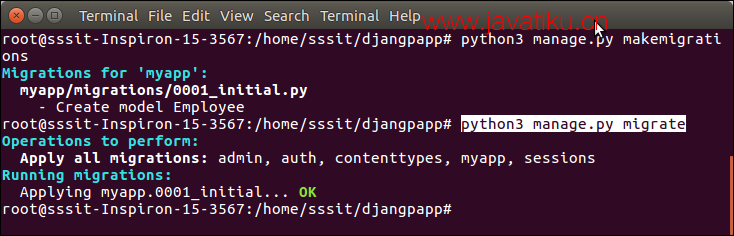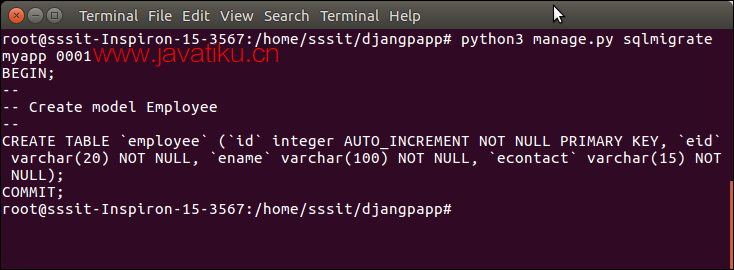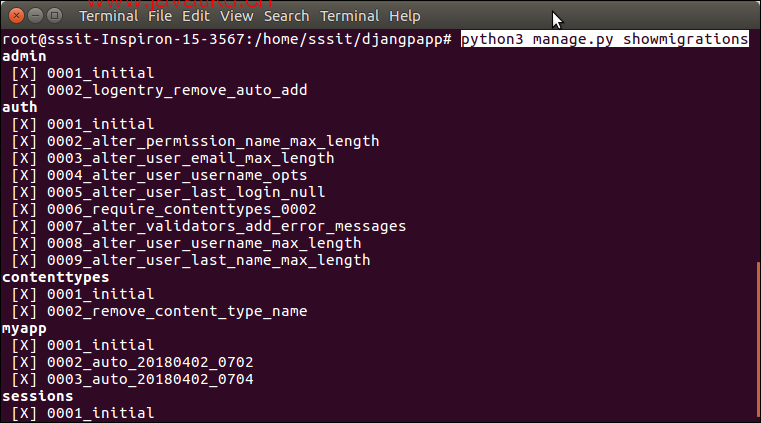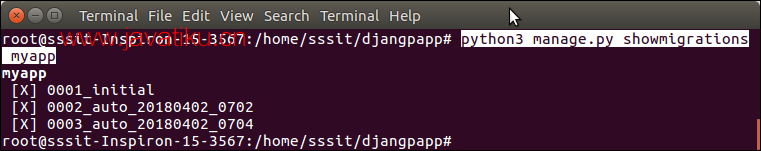# Diango教程-Django 数据库迁移Django 提供了用于执行迁移相关任务的各种命令。创建模型后，我们可以使用这些命令。

• makemigrations ：它用于创建包含模型表模式代码的迁移文件。
• migrate ：它根据迁移文件中定义的架构创建表。
• sqlmigrate ：用于显示所应用迁移的原始 SQL 查询。
• showmigrations ：它列出了所有迁移及其状态。

## 模型

//模型.py

``````from django.db import models
class Employee(models.Model):
eid = models.CharField(max_length=20)
ename = models.CharField(max_length=100)
econtact = models.CharField(max_length=15)
class Meta:
db_table = "employee"  ``````

``\$ python3 manage.py makemigrations  ``## 迁移

*// 0001_initial.py*

``````from django.db import migrations, models
class Migration(migrations.Migration):
initial = True
dependencies = [
]
operations = [
migrations.CreateModel(
name='Employee',
fields=[
('id', models.AutoField(auto_created=True, primary_key=True, serialize=False, verbose_name='ID')),
('eid', models.CharField(max_length=20)),
('ename', models.CharField(max_length=100)),
('econtact', models.CharField(max_length=15)),
],
options={
'db_table': 'employee',
},
),
]  ``````

``\$ python3 manage.py migrate  ````\$ python3 manage.py migrate  ``showmigrations 命令用于显示已应用的迁移。请参阅示例。

``\$ python3 manage.py showmigrations  ````\$ python3 manage.py showmigrations myapp ``